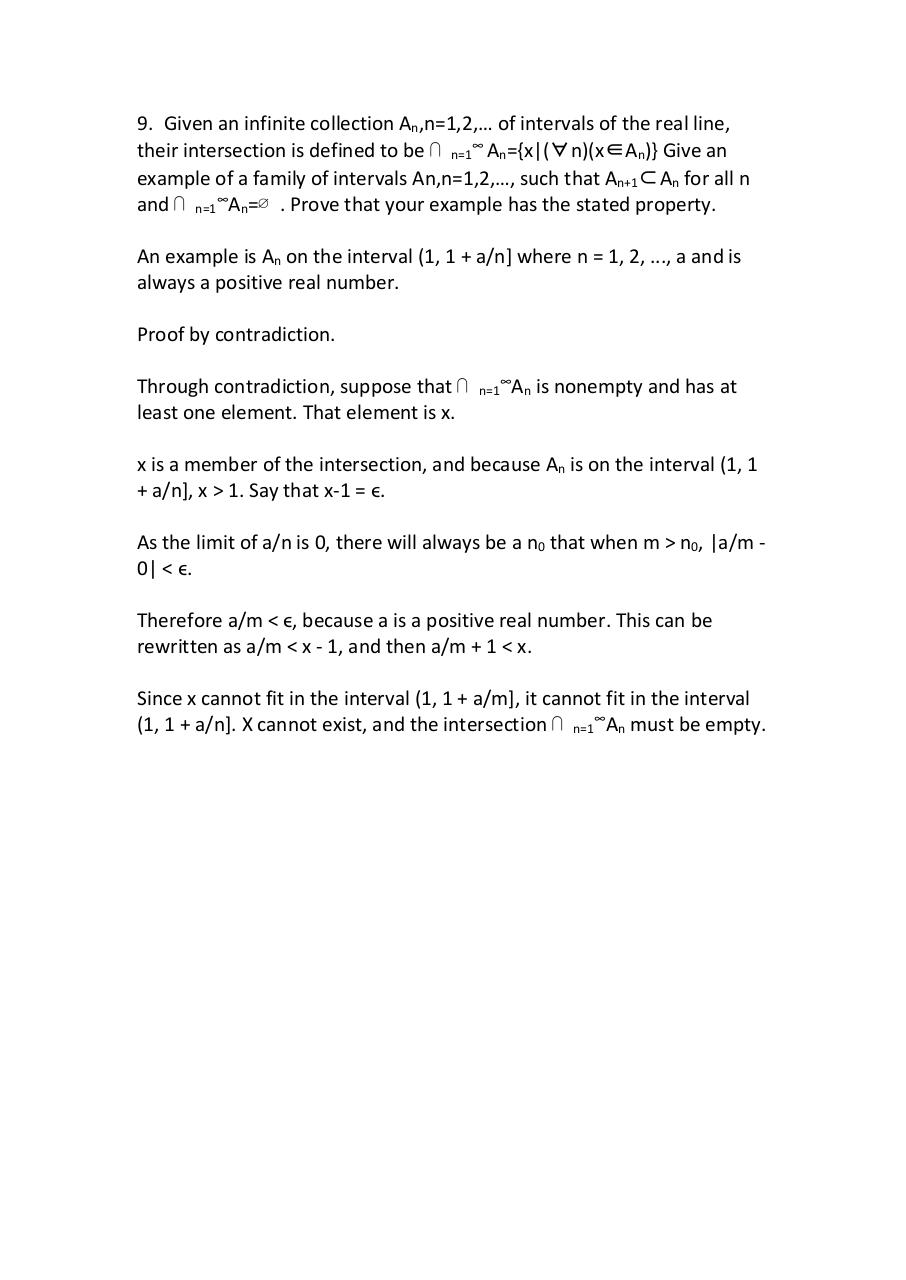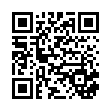proof9 .pdf

File information

Original filename: proof9.pdf
Author: vargh

This PDF 1.7 document has been generated by WPS Office / , and has been sent on pdf-archive.com on 28/08/2017 at 01:39, from IP address 108.218.x.x. The current document download page has been viewed 185 times.
File size: 74 KB (1 page).
Privacy: public file

proof9.pdf (PDF, 74 KB)

Document preview

9. Given an infinite collection An,n=1,2,… of intervals of the real line,
their intersection is defined to be ⋂ n=1∞ An={x|(∀n)(x∈An)} Give an
example of a family of intervals An,n=1,2,…, such that An+1⊂An for all n
and ⋂ n=1∞An=∅ . Prove that your example has the stated property.
An example is An on the interval (1, 1 + a/n] where n = 1, 2, ..., a and is
always a positive real number.
least one element. That element is x.

n=1

An is nonempty and has at

x is a member of the intersection, and because An is on the interval (1, 1
+ a/n], x &gt; 1. Say that x-1 = ϵ.
As the limit of a/n is 0, there will always be a n0 that when m &gt; n0, |a/m 0| &lt; ϵ.
Therefore a/m &lt; ϵ, because a is a positive real number. This can be
rewritten as a/m &lt; x - 1, and then a/m + 1 &lt; x.
Since x cannot fit in the interval (1, 1 + a/m], it cannot fit in the interval
(1, 1 + a/n]. X cannot exist, and the intersection ⋂ n=1∞An must be empty.HTML Code

Copy the following HTML code to share your document on a Website or Blog

QR CodeRelated keywords